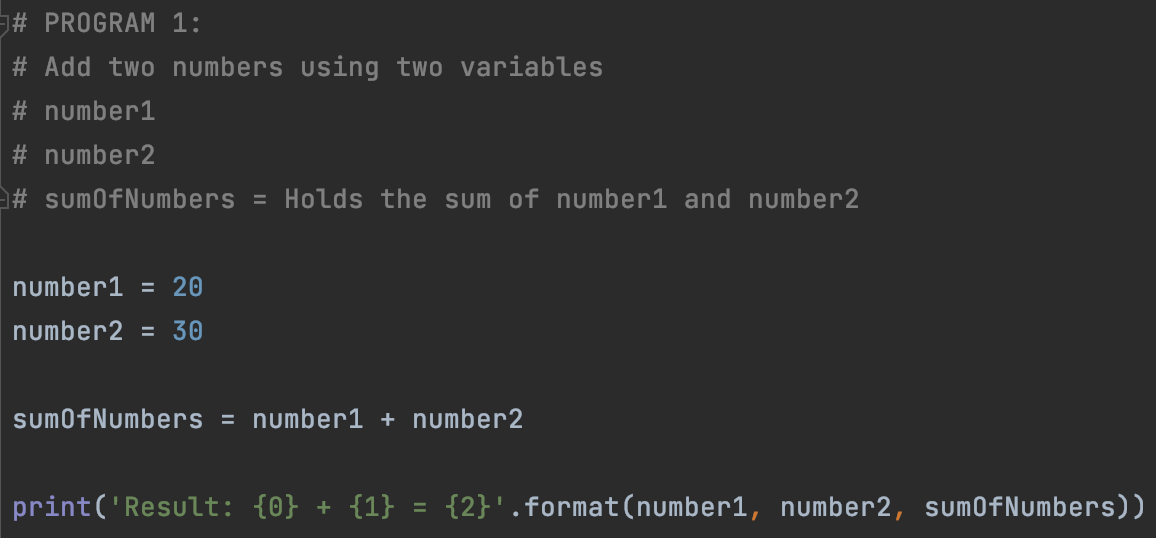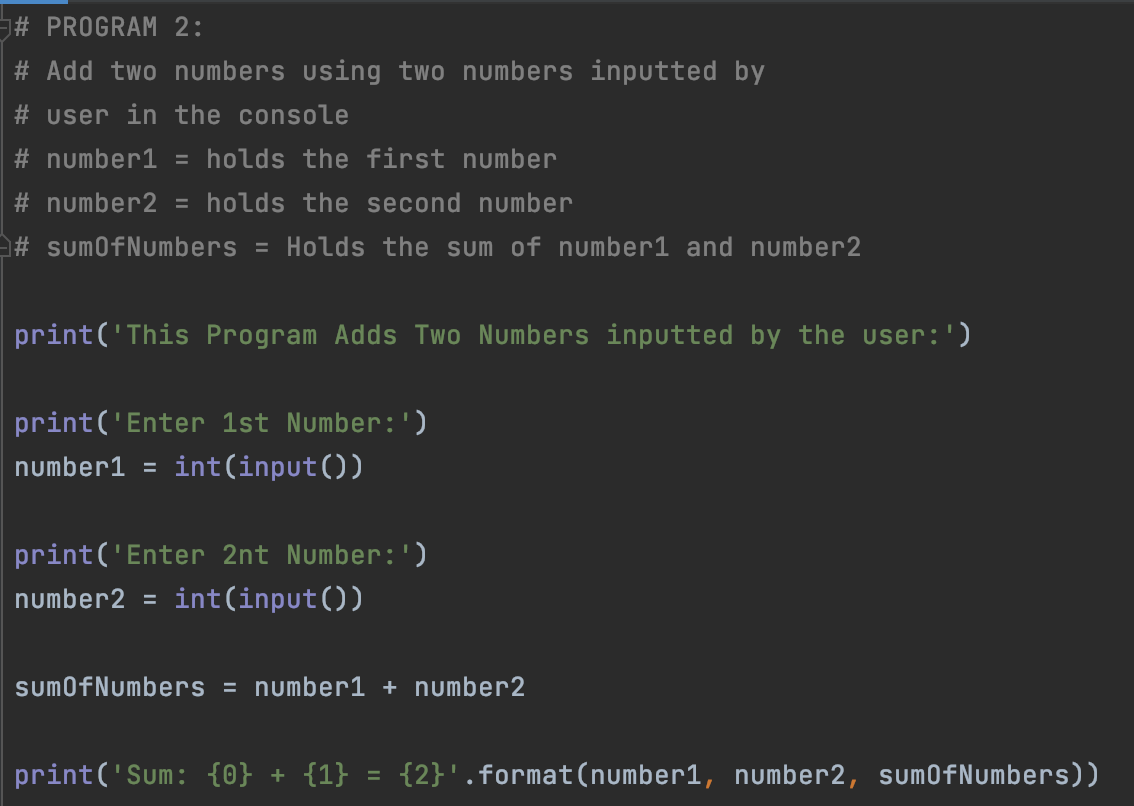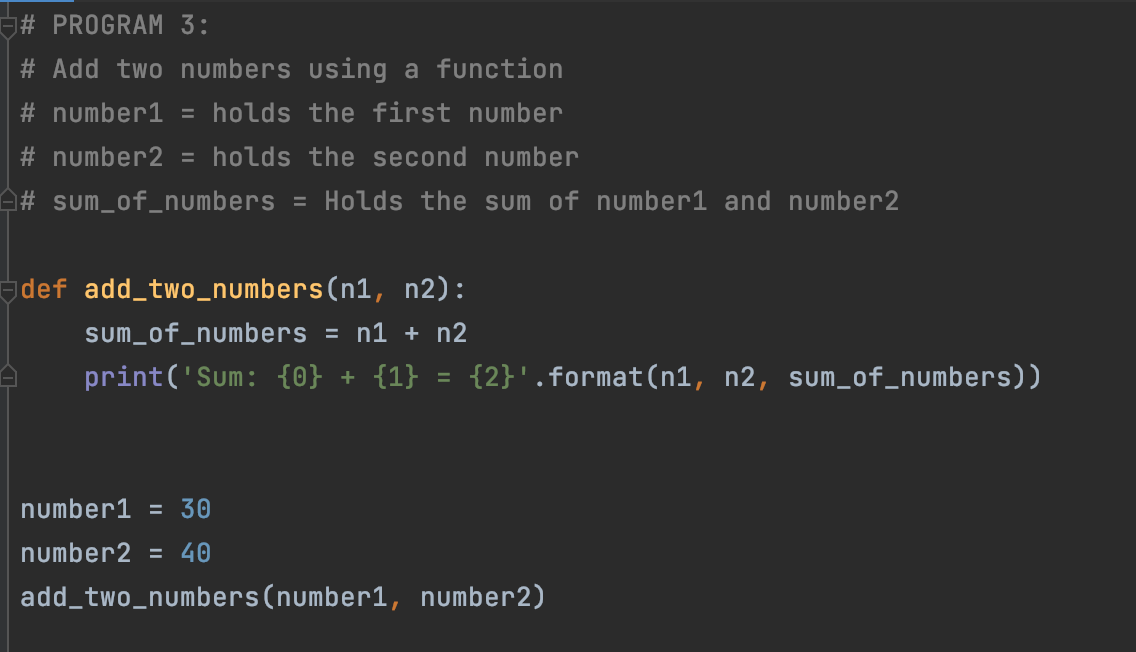# 3 Python program to add two numbers

Adding two numbers in Python can be done in various ways, we will be covering 3 ways in which you can write the same program to add two numbers,

``````
Add Two Numbers in Python using two variables.
Add Two Numbers in Python by numbers inputted by user in console.
Add Two Numbers in Pyhton using a function.
``````### Program 1: Add Two Numbers using two variables.

``````# PROGRAM 1:
# Add two numbers using two variables
# number1
# number2
# sumOfNumbers = Holds the sum of number1 and number2

number1 = 20
number2 = 30

sumOfNumbers = number1 + number2

print('Result: {0} + {1} = {2}'.format(number1, number2, sumOfNumbers))``````

Result: 20 + 30 = 50

### Program 2: Add Two Numbers by numbers inputted by user in console

``````# PROGRAM 2:
# Add two numbers using two numbers inputted by
# user in the console
# number1 = holds the first number
# number2 = holds the second number
# sumOfNumbers = Holds the sum of number1 and number2

print('This Program Adds Two Numbers inputted by the user:')

print('Enter 1st Number:')
number1 = int(input())

print('Enter 2nt Number:')
number2 = int(input())

sumOfNumbers = number1 + number2

print('Sum: {0} + {1} = {2}'.format(number1, number2, sumOfNumbers))``````

This Program Adds Two Numbers inputted by the user:
Enter 1st Number:
20
Enter 2nt Number:
80
Sum: 20 + 80 = 100

Process finished with exit code 0

### Program 3: Add Two Numbers by numbers inputted by user in console

``````# PROGRAM 3:
# Add two numbers using a function
# number1 = holds the first number
# number2 = holds the second number
# sum_of_numbers = Holds the sum of number1 and number2

sum_of_numbers = n1 + n2
print('Sum: {0} + {1} = {2}'.format(n1, n2, sum_of_numbers))

number1 = 30
number2 = 40